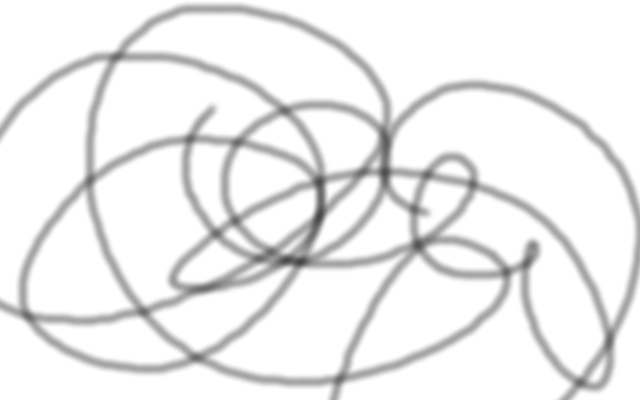# This is a markdown page

def f(x, y):
x = 5
print("asdf")
return t

str = """
asdfasdfasdf
"""


Here is some inline code

A list is:

• like
• this
• one

And then a block quote

here is a quote more of the quote

quote in a quote quoteception

And then

here is some inline math $\int_\epsilon^\infty e^{-ax}dx = 5$
$$\int_\epsilon^\infty e^{-ax}dx = 5$$